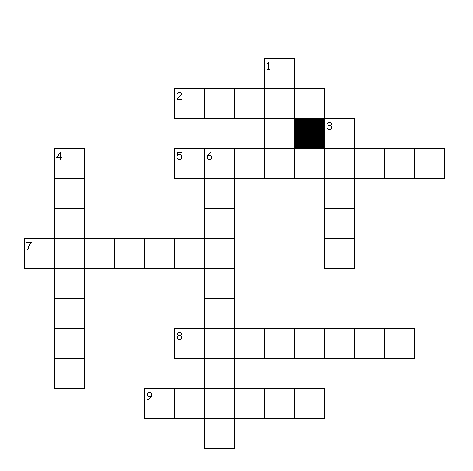# Important Questions for Work and Energy for Class 9 Science

Given below are the Class 9 Science Important Questions for work and energy
a. Concepts questions
b. Calculation problems
c. Multiple choice questions
e. Fill in the blank's

1. What is unit of energy?
2. The total energy when a body falls freely towards earth remains constant.  True or false?
3. What do you mean by kinetic energy of the body?
4. If the mass of the body is doubled and velocity is made halved, the kinetic energy will become?
5. Potential energy  is a vector quantity True or false?
6. When the force acts at right angle to the direction of motion, what is the work done by the force
7. What is Power?
8. What is Law of conservation of energy?

## Match the column

 Column A (Energy Conversion) Column B (Converters) Chemical energy into Mechanical energy Electric Generator Heat energy into Mechanical engine Electric Motor Mechanical energy into Electrical energy Car engine Electrical energy to Mechanical energy Steam engine Light energy into electrical energy Electric bulb Electrical energy into light energy Solar Cell

## Table type question

A force 10 N act on the body and body is displaced by 10 m. The angle between force and displacement are given below. Calculate the work done
 Angle 30 45 60 90 Work done ? ? ? ?

## Table type question

A body of mass 10 kg falls from the height 10 m towards the earth. Given g=10m/s2
 Height from earth(m) 10m ? ? 2m Potential energy(J) ? ? 500J ? Kinetic energy(J) ? 1000J ? ? Mechanical energy (J) ? ? ? ?

## Fill in the blanks

1. 1 Kilo Watt hour of energy is equal to ________ Joule
2. Power is the rate of doing ______. And its unit is ___________
3. Work done by the force can be _______ and ______.
4. The water stored in the reservoir of the tank possessed _______ energy
5. The total energy of the swinging pendulum remains ______ at all the points
6. When the body falls freely towards earth ,potential energy of the body _______while kinetic energy of the body______. The total remains ______at all the point during the motion

## Crossword PuzzleAcross
2. The scientist with whom unit of power is named
5. The kinetic energy of the body depends on mass and this quantity
7. The energy possessed by the car moving on the road
8. The Bigger unit of power
9. Work and power are these quantities
Down
1. The work done by the earth on the satellite moving in circular path
3. Solar water heater converts this energy into heat energy
4. The gravitation potential energy is present because of this
6. In hydroelectric power house, the potential energy of the water is finally transformed to this energy

Question 1.
An electric bulb of 100 W is used for 8 h per day. Calculate the 'units' of energy consumed in one day by the bulb?

Question 2.
Find the energy possessed by an object of mass 10 kg when it is at a height of 2 m above the ground. Given, g = 10 m/s2.

Question 3.
An engineer is asked to design a playground slide such that the speed a child reaches at the bottom does not exceed 4.0 m/s. Determine the maximum height that the slide can be.?Given, g = 9.8 m/s2.

Question 4.
Which one of the following is not the unit of energy?
(a) joule
(b) newton metre
(c) kilowatt
(d) kilowatt hour

Question 5.
A rocket is moving up with a velocity v. If the velocity of this rocket is suddenly tripled, what will be the ratio of two kinetic energies?

Question 6.
A force of 10 N displaces a body by a distance of 3 m at an angle 60° to its own direction. Find the amount of work done.

• Notes
• Assignment
• NCERT Solutions